• Call Now

1800-102-2727•

# Application of Newton's Second Law of Motion: How to Apply Newton's Second Law?

If there is a bike and a car in the parking lot, you are asked to move them by yourself. By intuition you will say that, you can not move a car by yourself. Now if I ask you how much force you need to apply to move any object having some mass with particular acceleration? Here Newton’s second law will help us out. In this article we will study the applications of Newton’s second law of motion. We will also deal with different types of problems that can be solved using the second law.

• How to Apply Newton's Second Law of Motion ?
• Practice Problems of Application of Newton's Second Law of Motion
• FAQs of Newton's Second Law of Motion

## Applications of Newton's Second Law of Motion

Newton’s second law of motion is applied when there is unbalanced force acting on an object. Here unbalanced force means that the resultant force on the object is not zero. The second law of motion is more quantitative and is used extensively to calculate what happens in situations involving a force. There are tremendous applications of the second law. Let’s take a look at some basic examples to understand:

### Kicking a Football:

When a player kicks a football, he exerts force in a specific direction. The stronger the football is kicked, the stronger the force he put on it and the further away it will travel.

A boy wants to kick a ball of mass 200 gram with an acceleration of 25 ms-2 then how much force he needs to apply on the ball? You can answer this easily by using the second law, F = m × a, so F = 0.2 × 25 = 5 N. So force of 5 N is required.### Pushing a Cart:

A boy pushing a grocery cart weighing 5 Kg with a force of 45 N as shown in the picture then by how much acceleration will the cart start moving? Again you can calculate the acceleration using the second law,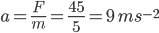. So the acceleration of the cart will be 9 ms-2.## How to Apply Newton's Second Law of Motion?

Normally any problem relating to Newton’s second law can be solved by using these steps:

1. First of all we decide the system on which the laws of motion are to be applied. The system may be a single particle, a block or combination of two or more blocks, two blocks connected by string, etc. The only restriction is that all parts of the system should have the same acceleration.
2. Once the system is decided, we make the list of all the forces acting on the system. Any force applied by the system on other bodies outside the system is not included in the list of forces.
3. Then we make the free body diagram of the system and indicate the magnitude and directions of all the forces and acceleration assigned to the body according to intuition listed in the above step in this diagram.
4. Then we choose any two mutually perpendicular axes X and Y in the plane of forces in case of coplanar forces. Choose the axis along the direction in which the system is known to have or is likely to have the acceleration.
5. Write the components of all the forces along the chosen axis and equate their vector sum to the product of the mass of the system and its acceleration in that direction
i.e.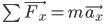and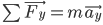.

## Practice Problems

Q1. A block of mass 5 Kg is kept on a smooth floor. A force of 3 N is applied on it at t=0. Find the acceleration of the block ?Solution: Here, as the floor is smooth, there is no friction. Thus applied force will cause mass to accelerate.

We know,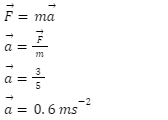Therefore the acceleration of block is 0.6 ms-2.

Q2. An arrangement of a two block system is shown. Find the net external force acting on a 1 kg and 2 kg block, respectively? (Assume the surfaces to be smooth)Solution: As both the blocks will move together with the applied force, acceleration of both the blocks will be the same.

Draw FBD:For First Block:

In the X direction, 6 - N12 = 1 × a ……….(1)

In the Y direction, N= m1g = 10 N

For Second Block:

In the X direction, N21 = 2a …………….(2)

In the Y direction, N= m2g = 20 N

[N12] = [N21]……….from third law

6=3a

a=2 ms-2

Net external force on 1 Kg is F = ma = 1 × 2 = 2 N

Net external force on 2 Kg is F = ma = 2 × 2 = 4 N

Q3. The body of mass 10 𝑘𝑔 is placed on a wedge (fixed to the ground) inclined at 60° to the horizontal and released. What is the magnitude of velocity of the block after 2 sec?Solution: FBD for the 10 kg block is as follows:Here, the x-axis is chosen along the downward inclined surface as the acceleration of the body will be along this direction.

mg sin60 = ma

a = g sin60

a = 53 ms-2

By using kinematic equations,

v = u + at

v = 0 + 53 × 2

v = 103 ms-1

Q4. The momentum of a particle moving in straight line is given as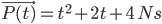. Find the force on a particle at t = 4 sec?

Solution: from newton's second law,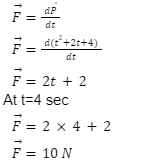Video Explanation: Application of second law

## FAQs

Question1. What is Newton’s second law of motion?
Newton’s second law of motion states that the rate of change of momentum of a body in a given inertial reference frame is directly proportional to the applied force and it takes place in the direction in which the force acts.

Question2. When do we apply Newton’s second law of motion?
The second law is more quantitative in nature and is used to analyse situations involving a force.

Question3. Can we use Newton’s second law of motion in a non-inertial frame of reference?
No, Newton’s laws are not valid in a non-inertial frame of reference. To use Newton's laws in a non-inertial frame you have to apply pseudo force.

Question4. What is the mathematical equation of the Newton's second law?
Mathematical formula of second law is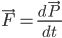.Talk to our expert
Resend OTP Timer =
By submitting up, I agree to receive all the Whatsapp communication on my registered number and Aakash terms and conditions and privacy policy